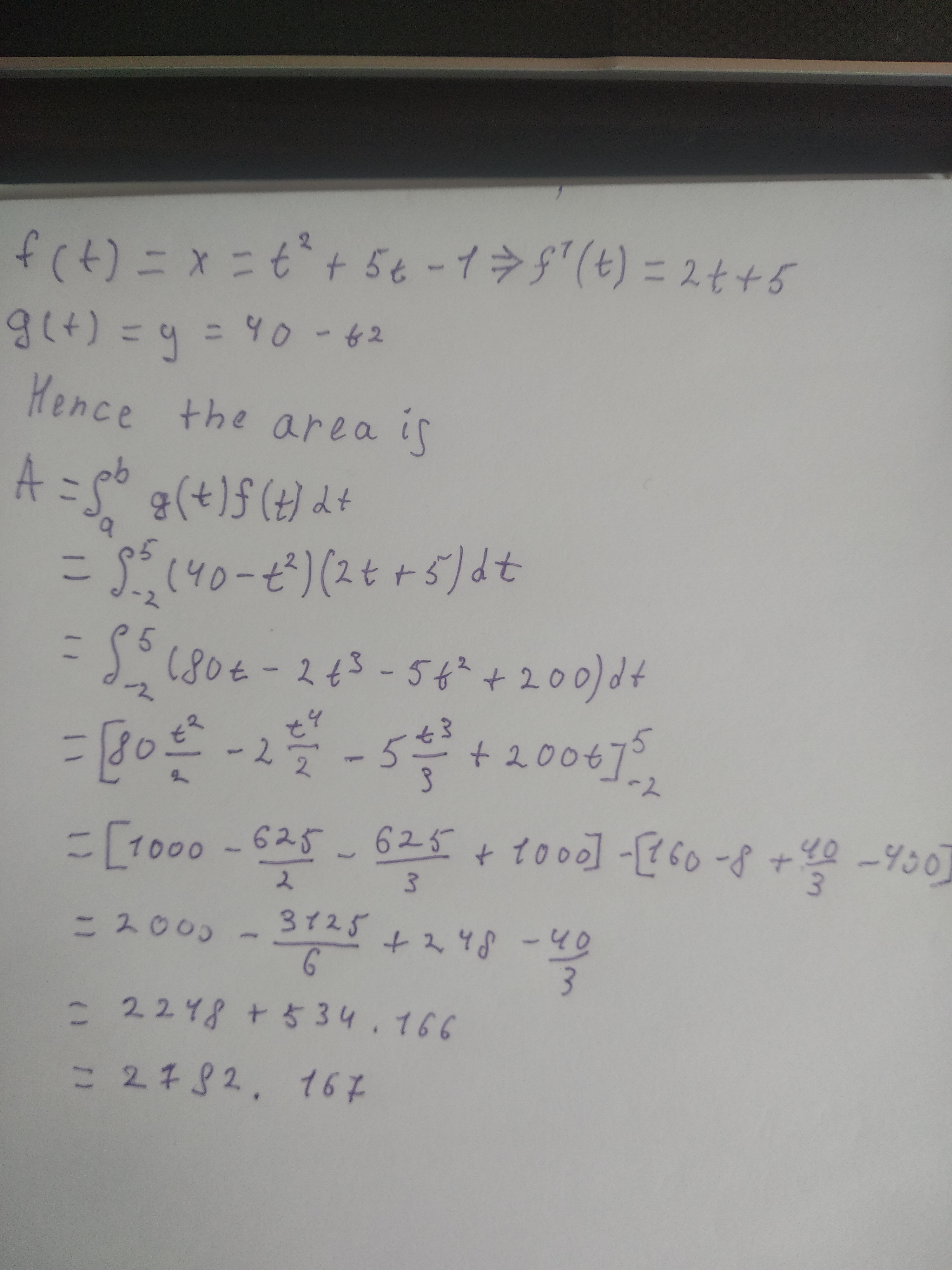Ask question

# Graph the plane curve defined by the parametric equations: x = t^2 + 1, y = 3t, -2 leq t leq 2.# Graph the plane curve defined by the parametric equations: x = t^2 + 1, y = 3t, -2 leq t leq 2.

Question
Parametric equations, polar coordinates, and vector-valued functionsasked 2021-03-09
Graph the plane curve defined by the parametric equations: $$x = t^2 + 1, y = 3t, -2 \leq t \leq 2$$.

## Answers (1)2021-03-10### Relevant Questionsasked 2021-02-24
Graph the plane curve defined by the parametric equations $$x = 125 cos t and y = 125 sin t$$asked 2020-11-06
Determine the area of the region below the parametric curve given by the set of parametric equations. For each problem you may assume that each curve traces out exactly once from right to left for the given range of t. For these problems you should only use the given parametric equations to determine the answer. 1.$$x = t^2 + 5t - 1 y = 40 - t^2 -2 \leq t \leq 5$$ 2.$$x = 3cos^2 (t) — sin^2 (t) y = 6 + cos(t) -\frac{\pi}{2} \neq t \leq 0$$ 3.$$x = e^{\frac{1}{4} t} —2 y = 4 + e^{\frac{1}{4 t}} — e^{\frac{1}{4} t} - 6 \leq t \leq 1$$asked 2021-03-01
Parametric equations and a value for the parameter t are given $$x = (60 cos 30^{\circ})t, y = 5 + (60 sin 30^{\circ})t - 16t2, t = 2$$. Find the coordinates of the point on the plane curve described by the parametric equations corresponding to the given value of t.asked 2021-01-08
Parametric to polar equations Find an equation of the following curve in polar coordinates and describe the curve. $$x = (1 + cos t) cos t, y = (1 + cos t) sin t, 0 \leq t \leq 2\pi$$asked 2021-03-05
1. A curve is given by the following parametric equations. x = 20 cost, y = 10 sint. The parametric equations are used to represent the location of a car going around the racetrack. a) What is the cartesian equation that represents the race track the car is traveling on? b) What parametric equations would we use to make the car go 3 times faster on the same track? c) What parametric equations would we use to make the car go half as fast on the same track? d) What parametric equations and restrictions on t would we use to make the car go clockwise (reverse direction) and only half-way around on an interval of [0, 2?]? e) Convert the cartesian equation you found in part “a” into a polar equation? Plug it into Desmos to check your work. You must solve for “r”, so “r = ?”asked 2021-02-21
Working with parametric equations Consider the following parametric equations. a. Eliminate the parameter to obtain an equation in x and y. b. Describe the curve and indicate the positive orientation. $$x=\ -7\ \cos\ 2t,\ y=\ -7\ \sin\ 2t,\ 0\ \leq\ t\ \leq\ \pi$$asked 2020-11-06
Write parametric, equations for the given curve for the given definitions $$y =\ -4x\ +\ 1$$ a) $$x = t$$ b) $$x=\ \frac{t}{2}$$ c) $$x =\ -4t$$asked 2021-02-12
Find parametric equations for the tangent line to the curve with the given parametric equations at the specified point. $$x=3\ \ln(t),\ y=4t^{\frac{1}{2}},\ z=t^{3},\ (0,\ 4,\ 1)$$asked 2020-10-27
That parametric equations contain more information than just the shape of the curve. Write a short paragraph explaining this statement. Use the following example and your answers to parts (a) and (b) below in your explanation. The position of a particle is given by the parametric equations $$x =\ \sin\ (t)\ and\ y =\ \cos\ (t)$$ where t represents time. We know that the shape of the path of the particle is a circle. a) How long does it take the particle to go once around the circle? Find parametric equations if the particle moves twice as fast around the circle. b) Does the particle travel clockwise or counterclockwise around the circle? Find parametric equations if the particle moves in the opposite direction around the circle.asked 2020-10-28
Consider the helix represented investigation by the vector-valued function $$r(t)=\ <\ 2\ \cos\ t,\ 2\ \sin\ t,\ t\ >$$. Solve for t in the relationship derived in part (a), and substitute the result into the original set of parametric equations. This yields a parametrization of the curve in terms of the arc length parameter s.
...